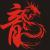## 向量空间 原

### 复数

C = {a + bi : a, b ∈ R}

### 向量空间

R2 = {(x, y) : x, y ∈ R}.这是我们熟悉的平面（plane）。R3 = {(x, y, z) : x, y, z ∈ R}.这是我们熟悉的立体空间（space）。

p(z) = a0 + a1z + a2z^2 + · · · + amz^m,p:F~F,是一个多项式的向量空间，很显然它并不是list，而是一个函数。

### 直和（direct sum）

The direct sum is an operation from abstract algebra, a branch of mathematics.

Suppose that U1, . . . , Un are subspaces of V. Then

V = U1 ⊕ · · · ⊕ Un if and only if both the following conditions hold:

(a) V = U1 + · · · + Un;

(b) the only way to write 0 as a sum u1 + · · · + un, where each

uj ∈ Uj, is by taking all the uj’s equal to 0.

### 评论(1)Essence of Linear Algebra

Essence of Linear Algebra 普适的代价是抽象 Abstractness is the price of generality 向量（Vector） 计算机学生眼里：向量是数字列表 物理学生眼里：向量是箭头 数学家认为：向量可以表示...

2018/12/05
0
0
AI之旅（3）：升维与最小二乘法

tieven
01/17
0
0
python_numpy_在二维三维空间中平面的正交基

Kedi
2016/04/19
0
0

2018/12/10
0
0

SVD
2015/12/04
62
0

Java单例模式学习记录

JerryLin123

1
0
VSCODE 无法调试

VSCODE 无法调试 可以运行 可能的原因： GCC 的参数忘了加 -g

shzwork

3
0

Tiny熊

4
0
5.线程实现

Eappo_Geng

3
0

Cobbage

5
0